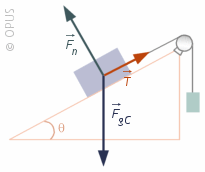## Tuesday, May 1, 2018

### Friction: A Force That Opposes Motion

Newton's Second Law leads to the formula that connects force, mass, and acceleration:

F = ma

Earlier, it was explained that there are only four fundamental forces, but that every other force that is easily observed is derived from them. Thus, friction can be understood as a force which arises due to the interaction of the atoms between two objects when they are in contact. The strong, weak, and electromagnetic forces work such that there is a resistive force when one object slides across another.

A resistive force (also know as oppositional force) works in the opposite direction of the active forces, such as pushing or pulling something.

Before working with friction, there are two more concepts that need to be understood:

### Weight

Weight is the force created by gravity and its magnitude can be found by multiplying the mass of an object by the rate at which gravity accelerates things. On earth, for introductory physics students,. the value for acceleration due to gravity of 9.8 (m/s)/s or 9.81 (m/s)/s is commonly used.

In the metric system, the Newton is the unit for weight. The pound is also a unit of weight*.

The direction for weight is always "down." That means that (when on earth) weight always acts in direction that is perpendicular to the horizon and toward the center of the earth.

### Normal ForceThe concept of Normal Force is easy to understand. Calculating the normal force (which is usually indicated as Fn) requires using trigonometry EXCEPT when the surface on which an object slides is level (has an angle to the horizon of zero).

The formal force (Fn) is defined as the component of the weight (mg) of an object that is perpendicular to a surface. When the surface is level ALL of the normal force is perpendicular to the surface. That makes things easy. Thus, the normal force IS THE WEIGHT, but this is ONLY true with the surface is level (has and angle to the horizon of zero).When the surface is NOT level, then, math…

Fn is labeled, and it is clear that it pushing the box in a direction that is perpendicular to the surface of the inclined plane.

### Note that F3 is perpendicular to the horizon. That would mean that F3 is the weight or is found as m•g where m is the mass and g is acceleration due to gravity.Force of Friction

There are two types of friction. One is when an object is already sliding. One is when the object is at rest. They are cleverly named sliding friction and static friction.

Sliding friction is sometimes indicated as fk where the k stands for kinetic (which means moving) and static friction is indicated as fs.

NOTE: in this nomenclature, the fi is lowercase. the k and the s may or may not be subscripted, depending on the type-setting process.

Intuition will tell you that pushing something over ice is easier than pushing it over asphalt. There therefore must be some way to indicate the degree of slipperiness an object has (or stickiness).

There is. It is called the coefficient of friction. And there are two sets of coefficients: one for each type of friction. Sliding and static coefficients of friction are abbreviated as µk and µs respectively.

Here is a link that will allow you to look at coefficients of friction for various materials:

https://www.engineeringtoolbox.com/friction-coefficients-d_778.html

### Calculating the Force of Friction

So, using all those concepts, calculating the force of friction becomes pretty simple. Friction can be found by multiplying the normal force by the coefficient of friction:

fk = (Fn)(µk)

EXAMPLE

Clean dry steel sliding on steel has a coefficient of friction of μ = 0.78. If a block with a mass of 4 kg slides across, what is the force of friction.

First, calculate the normal force:

Fn = mg
Fn = (4)(9.8)
Fn = 39.2

Now, use Fn to find the force of friction.

fk = (Fn)(µk)
fk = 39.2 • 0.78

fk = 30.576 N

_________________
*The English system unit for mass is called the Slug and is approximated by taking the weight of something and dividing by 32. So a 110 pound person would have a mass of 3.4 slugs.